# plot

Plot input and output channels of `iddata` object

## Syntax

``plot(data)``
``plot(data,LineSpec)``
``plot(data1,...,dataN)``
``plot(data1,LineSpec1...,dataN,LineSpecN)``
``plot(axes_handle,___)``
``plot(___,plotoptions)``
``h = plot(___)``

## Description

example

````plot(data)` plots the input and output channels of an `iddata` object. The function plots the outputs on the top axes and the inputs on the bottom axes.For time-domain data, the input and output signals are plotted as a function of time. Depending on the `InterSample` property of the `iddata` object, the input signals are plotted as linearly interpolated curves or as staircase plots. For example, if ```data.InterSample = 'zoh'```, the input is piecewise constant between sampling points, and is plotted accordingly.For frequency-domain data, the magnitude and phase of each input and output signal are plotted over the available frequency span.To plot a subset of the data, use subreferencing:`plot(data(201:300))` plots the samples 201 to 300 in the data set `data`.`plot(data(201:300,'Altitude',{'Angle_of_attack','Speed'}))` plots the specified samples of the output named `Altitude` and the inputs named `Angle_of_attack` and `Speed`.`plot(data(:,[3 4],[3:7]))` plots all samples of output channel numbers 3 and 4 and input numbers 3 through 7.```

example

````plot(data,LineSpec)` specifies the color, line style, and marker symbol for the dataset.```

example

````plot(data1,...,dataN)` plots multiple datasets. The number of plot axes is determined by the number of unique input and output names among all the datasets.```

example

````plot(data1,LineSpec1...,dataN,LineSpecN)` specifies the line style, marker type, and color for each dataset. You can specify options for only some data sets. For example, `plot(data1,data2,'k',data3)` specifies black as the plot color for `data2`.```

example

````plot(axes_handle,___)` plots into the axes with the handle `axes_handle` instead of into the current axes (`gca`). Use this syntax with any of the input argument combinations in the previous syntaxes.```

example

````plot(___,plotoptions)` specifies the plot options.```

example

````h = plot(___)` returns the handle to the plot. You can use this handle to customize the plot with `getoptions` and `setoptions`.```

## Examples

collapse all

`load iddata1 z1;`

Plot the data.

`plot(z1)`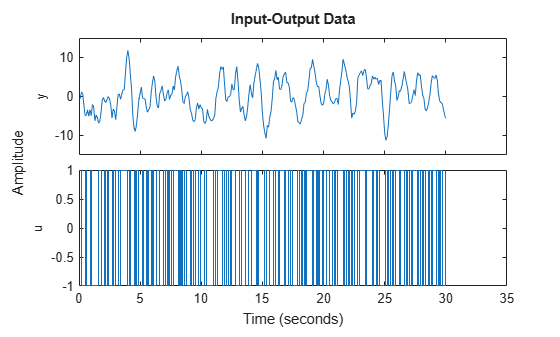The function plots the output on the top axes and the input on the bottom axes.

Plot the first 100 samples.

`plot(z1(1:100))`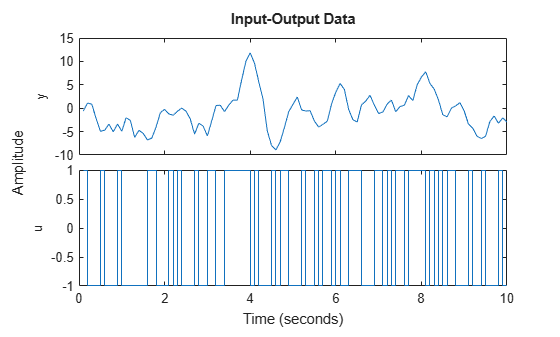Only the first 100 samples appear in the plot.

You can right-click the plot to explore characteristics such as peak and mean values.

`load iddata1 z1`

Convert the data to the frequency domain.

`zf = fft(z1);`

Plot the data.

`plot(zf);`Generate input data.

```u = idinput([100 1 20],'sine',[],[],[5 10 1]); u = iddata([],u,1,'per',100);```

Generate output data.

```sys = idtf(1,[1 2 1]); y = sim(sys,u);```

Plot only the input.

`plot(u)`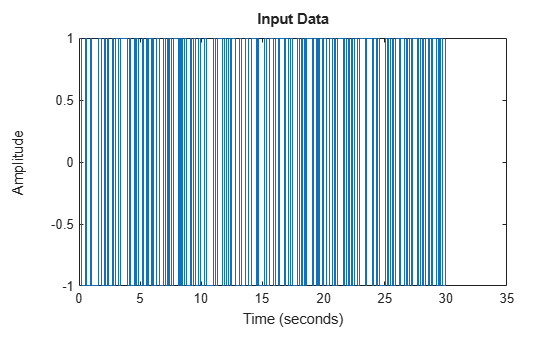Plot only the output.

`plot(y)`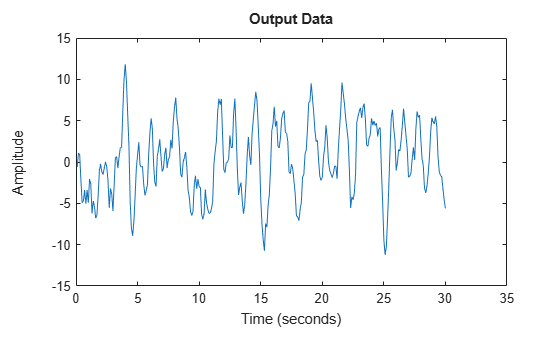Plot the input and output together.

`plot(y,u)`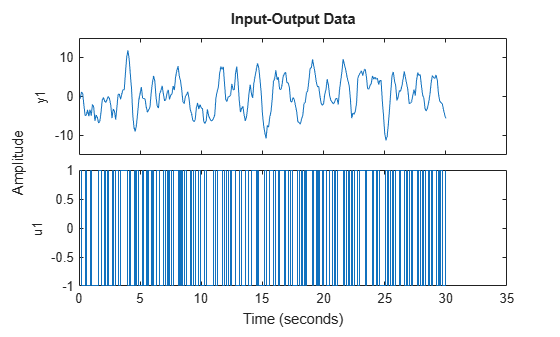Alternatively, you can use `plot(iddata(y,u))`.

```load iddata1 z1 load iddata2 z2```

Plot both datasets.

`plot(z1,z2)`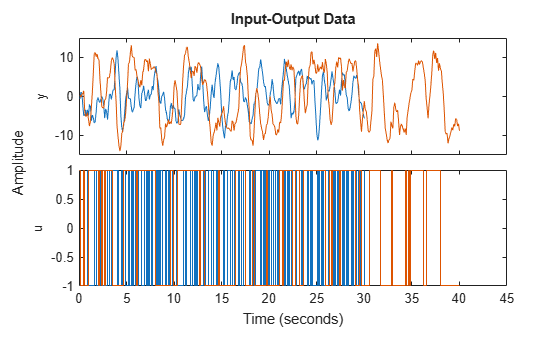Because the data sets use the same input and output names, the function plots both data sets together.

Specify unique input and output names.

```z1.InputName = "z1_input"; z2.InputName = "z2_input"; z1.OutputName = "z1_output"; z2.OutputName = "z2_output";```

Plot both datasets.

`plot(z1,z2)`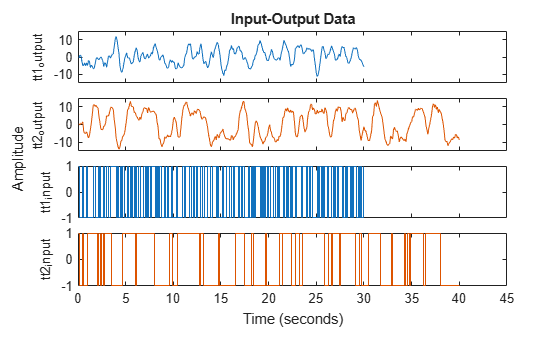The function plots the data sets separately.

Create a multiexperiment data set.

```load iddata1 z1 load iddata2 z2 zm = merge(z1,z2);```

Plot the data.

```plot(zm) legend('show')```For multiexperiment data, each experiment is treated as a separate data set. You can right-click the plots to view their characteristics.

```load iddata1 z1; load iddata2 z2;```

Specify the line style for both data sets.

`plot(z1,'y:*',z2,'b')`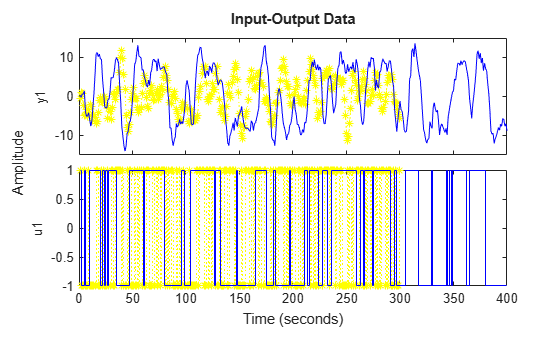Create a figure with two subplots and return the handles for each subplot axes in `s`.

```figure % new figure s(1) = subplot(1,2,1); % left subplot s(2) = subplot(1,2,2); % right subplot```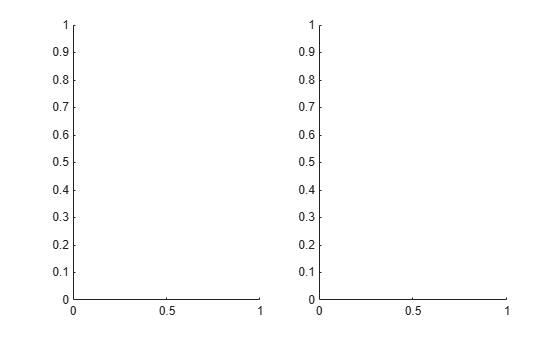```load iddata1; load iddata2;```

Create a data plot in each axes using the handles.

`plot(s(1),z1)``plot(s(2),z2)`Get the handle to your current plot and modify an axis property.

```load iddata1 z1 plot(z1)```Get the axes handle for the plot.

`ah = gca`
```ah = Axes (u1) with properties: XLim: [0.1000 30] YLim: [-1 1] XScale: 'linear' YScale: 'linear' GridLineStyle: '-' Position: [0.1300 0.1100 0.7750 0.3503] Units: 'normalized' Show all properties ```

The display shows the properties of the axes handle.

The scale of the x-axis `xScale` is `'linear'`. Change `xScale` to `'log'`.

`ah.XScale = 'log';`The x-axis now displays a log scale.

Configure a time plot.

`opt = iddataPlotOptions('time');`

Specify minutes as the time unit of the plot.

`opt.TimeUnits = 'minutes';`

Turn the grid on.

`opt.Grid = 'on';`

Create the plot with the options specified by `opt`.

```load iddata1 z1 plot(z1, opt);```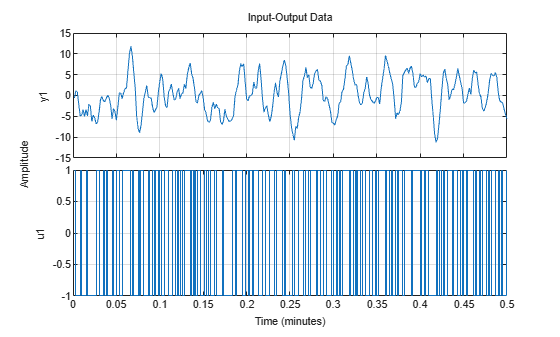Create a data plot and return the handle.

```load iddata1; h = plot(z1);```Set the time unit of the plot.

`setoptions(h,'TimeUnits','minutes');`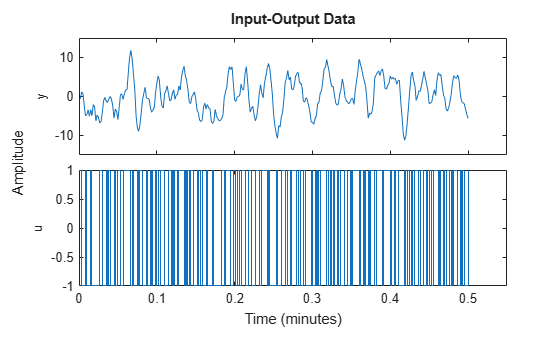Generate data with two inputs and one output.

`z = iddata(randn(100,1),rand(100,2));`

Configure a time plot.

`opt = iddataPlotOptions('time');`

Plot the data.

`h = plot(z,opt);`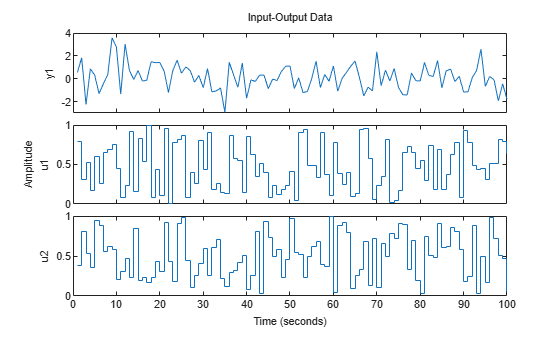Change the orientation of the plots such that all inputs are plotted in one column, and all outputs are in a second column.

```opt.Orientation = 'two-column'; h = plot(z,opt);```Alternatively, use `setoptions`.

`setoptions(h,'Orientation','two-column')`

You can also change the orientation by right-clicking the plot and choosing `Orientation` in the context menu.

## Input Arguments

collapse all

Input-output data, specified as an `iddata` object. The data can be in the time domain or the frequency domain. It can be a single-channel or multichannel data, and single-experiment or multiexperiment data.

Line style, marker symbol, and color, specified as a character vector. `LineSpec` takes values such as `'b'` and `'b+:'`. For more information, see the `plot` reference page in the MATLAB® documentation. For an example of using `LineSpec`, see Specify Line Style, Marker Symbol, and Color.

Axes handle, specified as a handle, and which is the reference to an `axes` object. Use the `gca` function to get the handle to the current axes, for example, `axes_handle= gca`. For an example of using `axes_handle` to apply a specific set of axes to the current plot, see Specify Axes Handle. For an example of using `gca` to get your plot axes and then modifying the axes properties, see Get and Use Axes Handle.

Plot options, specified as an option set created using `iddataPlotOptions`. For an example of using `plotoptions`, see Specify Plot Options.

## Output Arguments

collapse all

Plot handle, returned as a scalar or vector. Handles are unique identifiers that you can use to query and modify properties of a specific plot. For an example, see Change Plot Properties Using Handle.

## Tips

Right-clicking the plot opens the context menu, where you can access the following options and plot controls.

OptionDescription and Suboptions
DatasetsView the datasets used in the plot.
Characteristics

Peak Value — View the peak value of the data. This value is useful for transient data.

Mean Value — View the mean value of the data. This value is useful for steady-state data.

Orientation

For data with one input and one output channel:

• Single row — Plot all inputs and outputs in one row.

• Single column— Plot all inputs and outputs in one column.

For data with more than one input or output channel:

• Output row and input row — Plot all outputs in one row and all inputs in a second row.

• Output column and input column — Plot all outputs in one column and all inputs in a second column.

I/O Grouping

Group input and output channels on the plot.

Use this option with datasets with more than one input or output channel.

I/O Selector

Select a subset of the input and output channels to plot. By default, all input and output channels are plotted.

Use this option with data sets with more than one input or output channel.

NormalizeNormalize the y-scale of all data in the plot.
Properties Open the Property Editor dialog box, where you can customize plot attributes.